#The Fundamental Theorem of Algebra#### The multiplicity of roots.

Let's factor the polynomial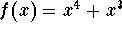. We can "pull out" a term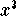: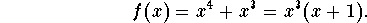Can we do anything else? No, we're done, we have factored the polynomial completely; indeed we have found the four linear (=degree 1) polynomials, which make up f(x):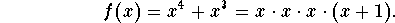It just happens that the linear factor x shows up three times. What are the roots of f(x)? There are two distinct roots: x=0 and x=-1. It is convenient to say in this situation that the root x=0 has multiplicity 3, since the term x=(x-0) shows up three times in the factorization of f(x). Of course, the other root x=-1 is said to have multiplicity 1.

We will from now on always count roots according to their multiplicity. So we will say that the polynomialhas FOUR roots.

Here is another example: How many roots does the polynomial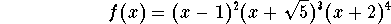have?

The root x=1 has multiplicity 2, the root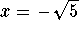has multiplicity 3, and the root x=-2 has multiplicity 4. All in all, the polynomial has 9 real roots!

A degree 2 polynomial is called a quadratic polynomial. In factoring quadratic polynomials, we naturally encounter three different cases:

Case 1A: Consider the quadratic polynomial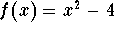depicted below.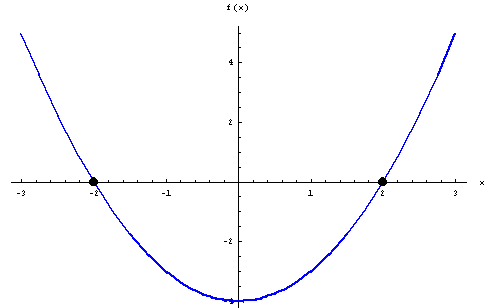The polynomial has 2 distinct real roots; indeed the two roots are x=2 and x=-2.

Case 1B: Consider the quadratic polynomial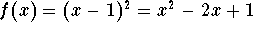depicted below.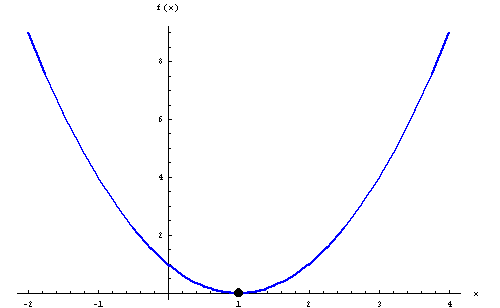The polynomial has only one x-intercept at x=1, but from the factoring we see that the root x=1 has multiplicity 2.

In both cases 1A and 1B, the quadratic polynomial has 2 real roots. Such quadratic polynomials are called reducible over the real numbers.

Case 2: Consider the quadratic polynomial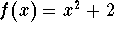depicted below.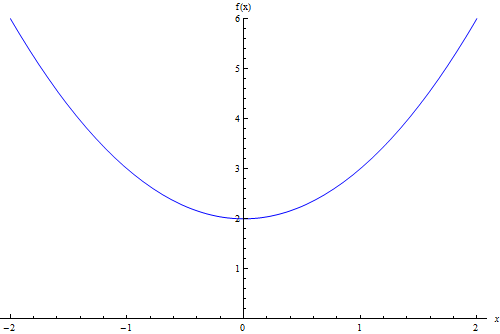The polynomial has no x-intercepts, consequently it has no real roots. (We will see later that it has 2 complex roots, though.)

In Case 2, the quadratic polynomial has no real roots. Such a quadratic polynomial is called irreducible over the real numbers.

At this point, the easiest way to tell, whether a quadratic polynomial is reducible or irreducible, is to graph it.

#### The Fundamental Theorem of Algebra.

It turns out that linear factors (=polynomials of degree 1) and irreducible quadratic polynomials are the "atoms", the building blocks, of all polynomials:

 Every polynomial can be factored (over the real numbers) into a product of linear factors and irreducible quadratic factors.The Fundamental Theorem of Algebra was first proved by Carl Friedrich Gauss (1777-1855).

What does The Fundamental Theorem of Algebra tell us? It tells us, when we have factored a polynomial completely:

On the one hand, a polynomial has been completely factored (over the real numbers) only if all of its factors are linear or irreducible quadratic.

On the other hand, whenever a polynomial has been factored into only linear and irreducible quadratics, then it has been factored completely, since both linear factors and irreducible quadratics cannot be factored any further over the real numbers.

What does The Fundamental Theorem of Algebra not tell us? It is not constructive, that is, it does not tell us how to factor a polynomial completely!In fact, it is not known in general how to factor a polynomial; only techniques for special kinds of polynomials are known. It is even worse than that: The mathematician Evariste Galois (1811-1832) has proved that there will never be a general formula to solve polynomials of degree 5 and higher.

#### Uniqueness of factorization.

Is factorization unique? Yes, and No! If a polynomial has leading coefficient 1, then its factorization is unique, as long as we require all factors to have leading coefficient 1. Consider the example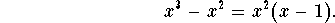The polynomial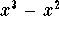has leading coefficient 1, so do the factors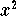and x-1. This is the only way to factor, unless we "split hairs" and consider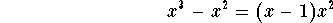as a different factorization.

On the other hand, if the leading coefficient is not equal to 1, there are slight problems: Consider the example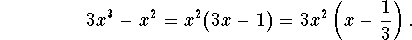Which of these two factorizations is better? I do not know, but we can fix this problem and regain uniqueness, if we insist on the following: all factors have leading coefficient 1, and the leading coefficient of the original polynomial is written in front of the factors: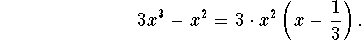With this agreement, factorization becomes unique again.

Another example: factor the polynomial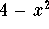. The leading coefficient is -1; the standard factorization would be written as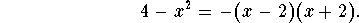#### Exercise 1.

Find all real roots and their multiplicity of the polynomial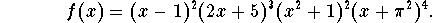#### Exercise 2.

Is the following quadratic polynomial reducible or irreducible?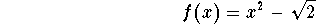#### Exercise 3.

Is the following quadratic polynomial reducible or irreducible?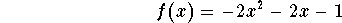#### Exercise 4.

Factor completely: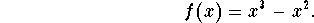#### Exercise 5.

Factor completely: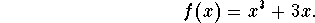[Back] [Next]
[Algebra] [Trigonometry] [Complex Variables]
[Calculus] [Differential Equations] [Matrix Algebra]S.O.S MATHematics home page

Do you need more help? Please post your question on our S.O.S. Mathematics CyberBoard.Helmut Knaust